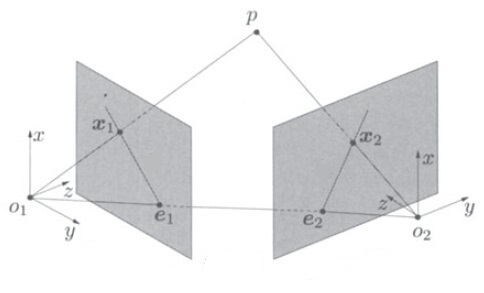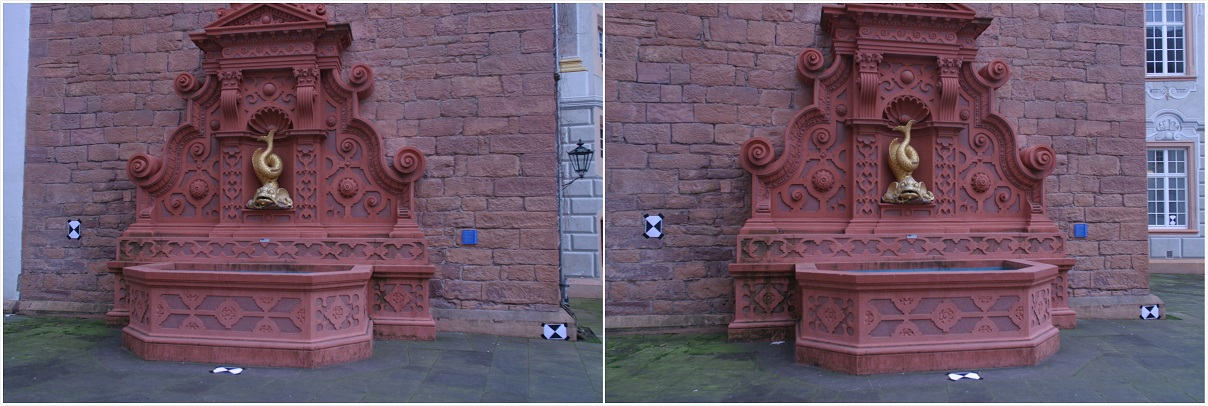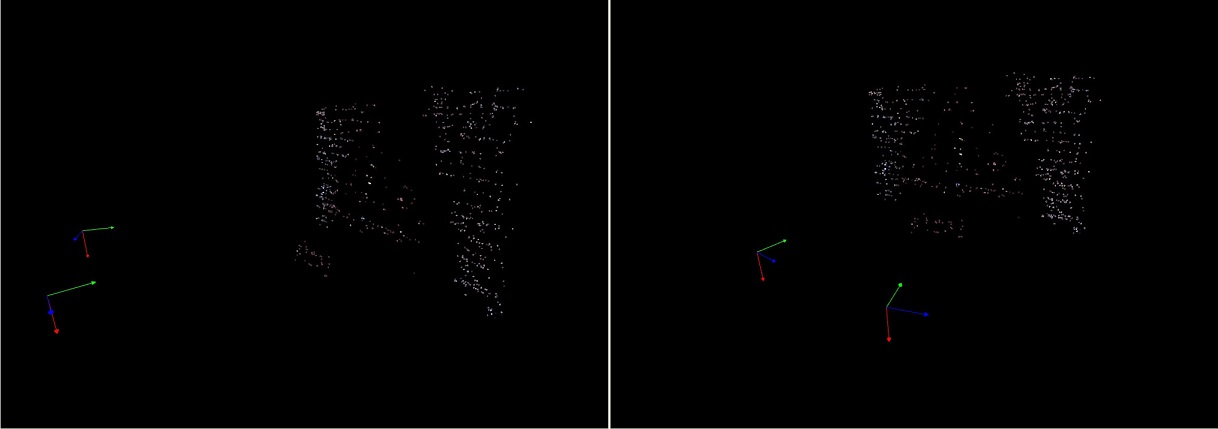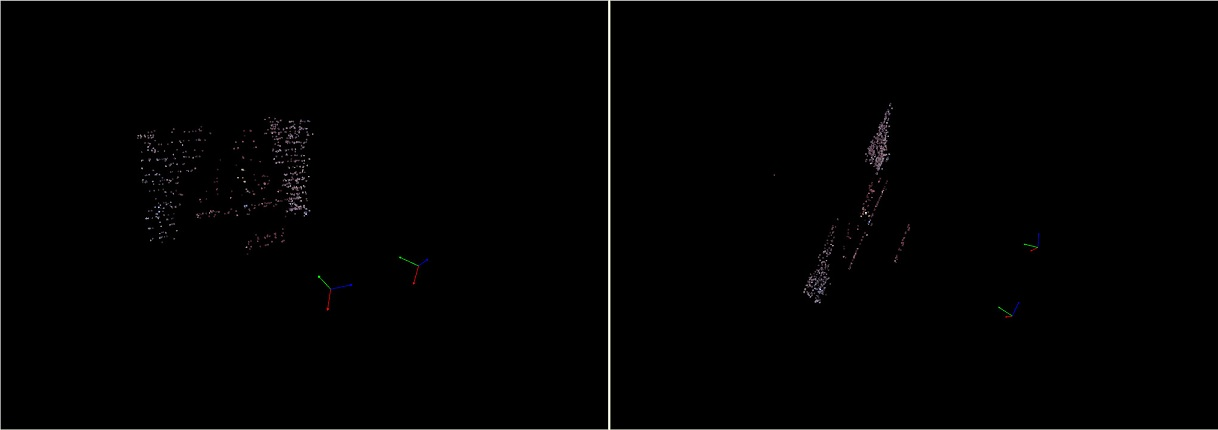# OpenCV实现SfM（二）：双目三维重建

### 文章目录

#极线约束与本征矩阵X X 到两个相机像面的垂直距离分别为 s 1 s_1 s 2 s_2 ，且这两个相机具有相同的内参矩阵 K K ，与世界坐标系之间的变换关系分别为 [ R 1    T 1 ] [R_1\ \ T_1] [ R 2    T 2 ] [R_2\ \ T_2] ，那么我们可以得到下面两个等式
s 1 x 1 = K ( R 1 X + T 1 ) s 2 x 2 = K ( R 2 X + T 2 ) s_1x_1 = K(R_1X + T_1) \\ s_2x_2 = K(R_2X + T_2)

s 1 K − 1 x 1 = R 1 X + T 1 s 2 K − 1 x 2 = R 2 X + T 2 s_1K^{-1}x_1 = R_1X + T_1 \\ s_2K^{-1}x_2 = R_2X + T_2
K − 1 x 1 = x 1 ′ K^{-1}x_1 = x_1^{'} K − 1 x 2 = x 2 ′ K^{-1}x_2 = x_2^{'} ，则有
s 1 x 1 ′ = R 1 X + T 1 s 2 x 2 ′ = R 2 X + T 2 s_1x_1^{'} = R_1X + T_1 \\ s_2x_2^{'} = R_2X + T_2

s 1 x 1 ′ = X s 2 x 2 ′ = R 2 X + T 2 s_1x_1^{'} = X \\ s_2x_2^{'} = R_2X + T_2

s 2 x 2 ′ = s 1 R 2 x 1 ′ + T 2 s_2x_2^{'} = s_1R_2x_1^{'} + T_2
x 2 ′ x_2^{'} T 2 T_2 都是三维向量，它们做外积（叉积）之后得到另外一个三维向量 T 2 ^ x 2 ′ \widehat{T_2}x_2^{'} （其中 T 2 ^ \widehat{T_2} 为外积的矩阵形式， T 2 ^ x 2 ′ \widehat{T_2}x_2^{'} 代表 T 2 × x 2 ′ T_2\times x_2^{'} ），且该向量垂直于 x 2 ′ x_2^{'} T 2 T_2 ，再用该向量对等式两边做内积，有
0 = s 1 ( T 2 ^ x 2 ′ ) T R 2 x 1 ′ 0 = s_1(\widehat{T_2}x_2^{'})^TR_2x_1^{'}

x 2 ′ T 2 ^ R 2 x 1 ′ = 0 x_2^{'}\widehat{T_2}R_2x_1^{'} = 0
E = T 2 ^ R 2 E = \widehat{T_2}R_2
x 2 ′ E x 1 ′ = 0 x_2^{'}Ex_1^{'} = 0

#特征点提取与匹配

void extract_features(
vector<string>& image_names,
vector<vector<KeyPoint>>& key_points_for_all,
vector<Mat>& descriptor_for_all,
vector<vector<Vec3b>>& colors_for_all
)
{
key_points_for_all.clear();
descriptor_for_all.clear();
Mat image;

//读取图像，获取图像特征点，并保存
Ptr<Feature2D> sift = xfeatures2d::SIFT::create(0, 3, 0.04, 10);
for (auto it = image_names.begin(); it != image_names.end(); ++it)
{
if (image.empty()) continue;

vector<KeyPoint> key_points;
Mat descriptor;
//偶尔出现内存分配失败的错误
sift->detectAndCompute(image, noArray(), key_points, descriptor);

//特征点过少，则排除该图像
if (key_points.size() <= 10) continue;

key_points_for_all.push_back(key_points);
descriptor_for_all.push_back(descriptor);

vector<Vec3b> colors(key_points.size());
for (int i = 0; i < key_points.size(); ++i)
{
Point2f& p = key_points[i].pt;
colors[i] = image.at<Vec3b>(p.y, p.x);
}
colors_for_all.push_back(colors);
}
}

void match_features(Mat& query, Mat& train, vector<DMatch>& matches)
{
vector<vector<DMatch>> knn_matches;
BFMatcher matcher(NORM_L2);
matcher.knnMatch(query, train, knn_matches, 2);

//获取满足Ratio Test的最小匹配的距离
float min_dist = FLT_MAX;
for (int r = 0; r < knn_matches.size(); ++r)
{
//Ratio Test
if (knn_matches[r].distance > 0.6*knn_matches[r].distance)
continue;

float dist = knn_matches[r].distance;
if (dist < min_dist) min_dist = dist;
}

matches.clear();
for (size_t r = 0; r < knn_matches.size(); ++r)
{
//排除不满足Ratio Test的点和匹配距离过大的点
if (
knn_matches[r].distance > 0.6*knn_matches[r].distance ||
knn_matches[r].distance > 5 * max(min_dist, 10.0f)
)
continue;

//保存匹配点
matches.push_back(knn_matches[r]);
}
}


bool find_transform(Mat& K, vector<Point2f>& p1, vector<Point2f>& p2, Mat& R, Mat& T, Mat& mask)
{
//根据内参矩阵获取相机的焦距和光心坐标（主点坐标）
double focal_length = 0.5*(K.at<double>(0) + K.at<double>(4));
Point2d principle_point(K.at<double>(2), K.at<double>(5));

//根据匹配点求取本征矩阵，使用RANSAC，进一步排除失配点
Mat E = findEssentialMat(p1, p2, focal_length, principle_point, RANSAC, 0.999, 1.0, mask);
if (E.empty()) return false;

cout << (int)feasible_count << " -in- " << p1.size() << endl;
//对于RANSAC而言，outlier数量大于50%时，结果是不可靠的
if (feasible_count <= 15 || (feasible_count / p1.size()) < 0.6)
return false;

//分解本征矩阵，获取相对变换
int pass_count = recoverPose(E, p1, p2, R, T, focal_length, principle_point, mask);

//同时位于两个相机前方的点的数量要足够大
if (((double)pass_count) / feasible_count < 0.7)
return false;

return true;
}


#三维重建

s 2 x 2 = K ( R 2 X + T 2 ) s_2x_2 = K(R_2X + T_2)

0 = x 2 ^ K ( R 2 X + T 2 ) 0 = \widehat{x_2}K(R_2X+T_2)

x 2 ^ K R 2 X = − x 2 ^ K T 2 \widehat{x_2}KR_2X = -\widehat{x_2}KT_2

x 2 ^ K ( R 2    T ) ( X 1 ) = 0 \widehat{x_2}K(R_2\ \ T)\left(\begin{matrix}X \\ 1\end{matrix}\right) = 0

void reconstruct(Mat& K, Mat& R, Mat& T, vector<Point2f>& p1, vector<Point2f>& p2, Mat& structure)
{
//两个相机的投影矩阵[R T]，triangulatePoints只支持float型
Mat proj1(3, 4, CV_32FC1);
Mat proj2(3, 4, CV_32FC1);

proj1(Range(0, 3), Range(0, 3)) = Mat::eye(3, 3, CV_32FC1);
proj1.col(3) = Mat::zeros(3, 1, CV_32FC1);

R.convertTo(proj2(Range(0, 3), Range(0, 3)), CV_32FC1);
T.convertTo(proj2.col(3), CV_32FC1);

Mat fK;
K.convertTo(fK, CV_32FC1);
proj1 = fK*proj1;
proj2 = fK*proj2;

//三角化重建
triangulatePoints(proj1, proj2, p1, p2, structure);
}


#测试04-2407-044305
09-301090
11-141万+
01-201万+
10-021万+
10-307202
11-244011
04-307843
09-013万+
06-195348
05-071万+
07-272万+
09-191万+
06-14185
08-081747
12-08